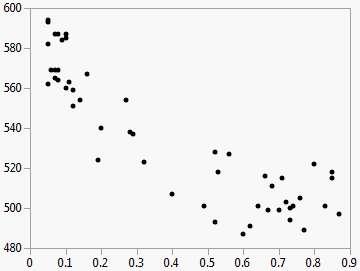Basic Analysis > Bivariate Analysis
Publication date: 07/30/2020

# Bivariate Analysis

##### Examine Relationships between Two Continuous Variables

The Bivariate platform shows the relationship between two continuous variables. It is the continuous by continuous personality of the Fit Y by X platform. The word bivariate simply means involving two variables instead of one (univariate) or many (multivariate).

The Bivariate analysis results appear in a scatterplot. Each point on the plot represents the X and Y values for a single observation. In other words, each point represents two variables. Using the scatterplot, you can see at a glance the degree and pattern of the relationship between the two variables. You can interactively add other types of fits, such as simple linear regression, polynomial regression, and so on.

Figure 5.1 Example of Bivariate Analysis